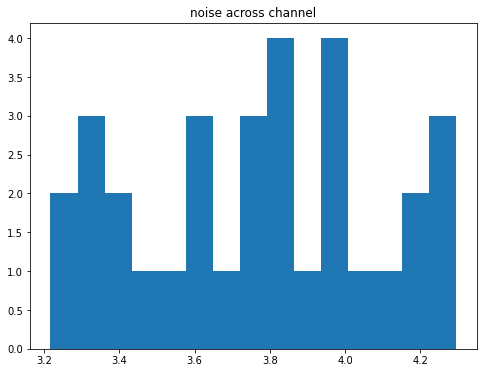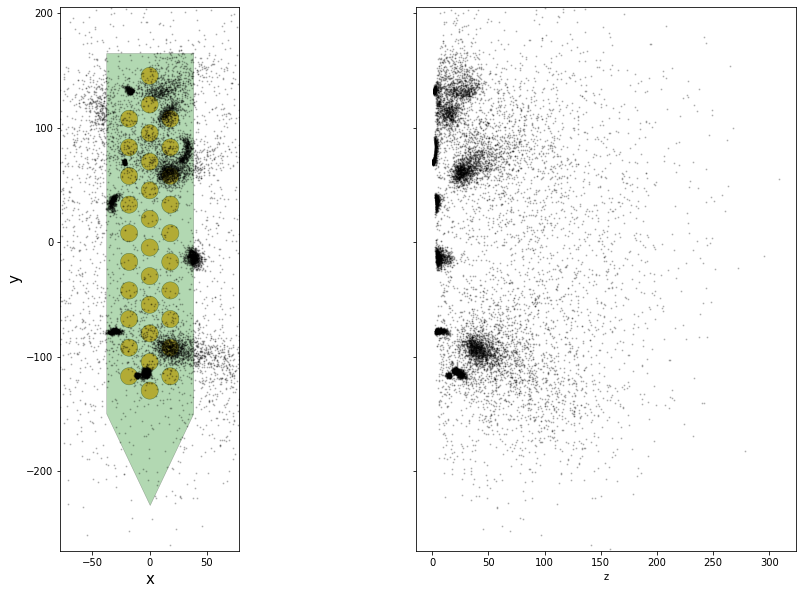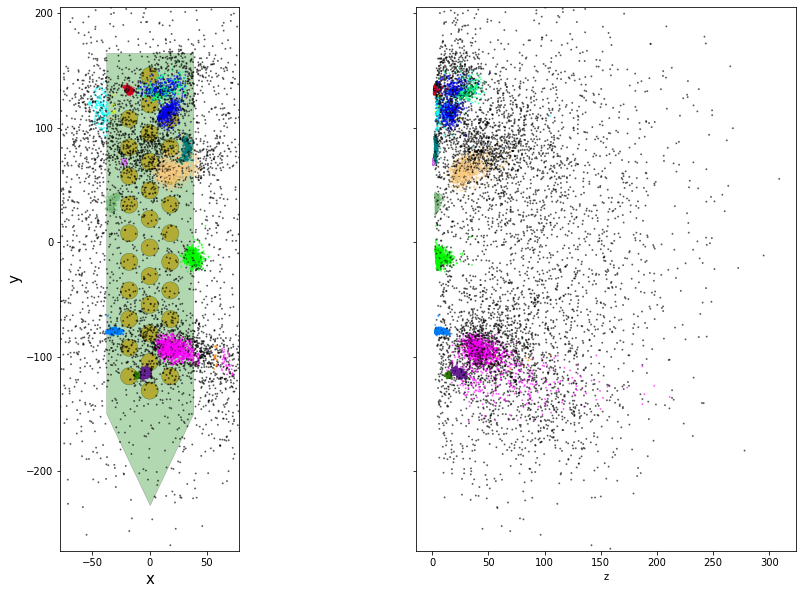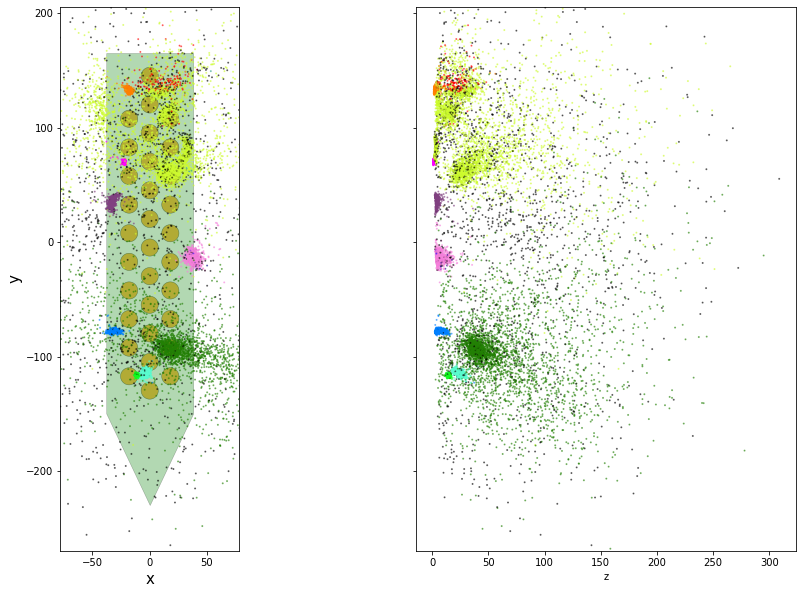# spikeinterface clustering

## spikeinterface clustering¶

The clustering step remains the central step of the spike sorting. Historically this step was separted into two distinct parts: feature reduction and clustering. In spikeinterface, we decided to regroup this two steps in the same module. This allows one to compute feature reduction on-the-fly and avoid long computations and storage of large features.

The clustering step takes the recording and detected (and optionally selected) peaks as input and returns a label for every peak.

At the moment, the implemenation is quite experimental. These methods have been implemented:

• "position_clustering": use HDBSCAN on peak locations.
• "sliding_hdbscan": clustering approach from tridesclous, with sliding spatial windows. PCA and HDBSCAN are run on local/sparse waveforms.
• "position_pca_clustering": this method tries to use peak locations for a first clustering step and then perform further splits using PCA + HDBSCAN

Different methods may need different inputs (for instance some of them require need peak locations and some do not).

For this we will use a simulated with mearec dataset on 32 channel neuronexus like probe.

Here we will also use the select_peak() function to sub sample a smaller number of peak

In :
# %matplotlib widget
%matplotlib inline

In :
%load_ext autoreload

In :
from pathlib import Path

import time

import numpy as np
import matplotlib.pyplot as plt

import spikeinterface.full as si

/home/samuel.garcia/.virtualenvs/py38/lib/python3.8/site-packages/datalad/cmd.py:375: RuntimeWarning: coroutine 'run_async_cmd' was never awaited
new_loop = True
RuntimeWarning: Enable tracemalloc to get the object allocation traceback
/home/samuel.garcia/Documents/SpikeInterface/spikeinterface/spikeinterface/core/datasets.py:12: RuntimeWarning: coroutine 'run_async_cmd' was never awaited
RuntimeWarning: Enable tracemalloc to get the object allocation traceback

In :
base_folder = Path('/mnt/data/sam/DataSpikeSorting/mearec_template_matching')
mearec_file = base_folder / 'recordings_collision_15cells_Neuronexus-32_1800s.h5'
rec_folder = base_folder /'Preprocessed_recording_15cells_Neuronexus-32_1800s'
peak_folder = base_folder / 'Peak_recording_15cells_Neuronexus'

clustering_path = base_folder / 'Clustering_recording_15cells_Neuronexus'

peak_folder.mkdir(exist_ok=True)

In :
# global kwargs for parallel computing
job_kwargs = dict(
n_jobs=40,
chunk_duration='1s',
progress_bar=True,
)


#### Preprocess¶

In :
# load already cache or compute
if rec_folder.exists():
else:
recording = si.bandpass_filter(recording, dtype='float32')
recording = si.common_reference(recording)
rec_preprocessed = recording.save(folder=rec_folder, n_jobs=20, chunk_size=30000, progress_bar=True)


#### estimate noise¶

In :
noise_levels = si.get_noise_levels(rec_preprocessed, return_scaled=False)
fig, ax = plt.subplots(figsize=(8,6))
ax.hist(noise_levels, bins=15)
ax.set_title('noise across channel')

Out:
Text(0.5, 1.0, 'noise across channel')### detect peaks¶

In :
from spikeinterface.sortingcomponents.peak_detection import detect_peaks

In :
if not (peak_folder / 'peaks.npy').exists():
peaks = detect_peaks(
rec_preprocessed,
method='locally_exclusive',
peak_sign='neg',
detect_threshold=5,
n_shifts=10,
noise_levels=noise_levels,
**job_kwargs,
)
np.save(peak_folder / 'peaks.npy', peaks)
print(peaks.shape)

(271519,)


### select peaks¶

In :
from spikeinterface.sortingcomponents.peak_selection import select_peaks

In :
if not (peak_folder / 'some_peaks.npy').exists():
some_peaks = select_peaks(peaks, method='uniform', select_per_channel=True, n_peaks=500, seed=None)
np.save(peak_folder / 'some_peaks.npy', some_peaks)
print('some_peaks.size', some_peaks.size)

some_peaks.size 13424


### localize peaks (on sub selection)¶

In :
from spikeinterface.sortingcomponents.peak_localization import localize_peaks

In :
some_peak_locations = localize_peaks(rec_preprocessed, some_peaks,
method='monopolar_triangulation',
method_kwargs={'local_radius_um': 100., 'max_distance_um': 1000., 'optimizer': 'minimize_with_log_penality'},
# method_kwargs={'local_radius_um': 100., 'max_distance_um': 1000., 'optimizer': 'least_square'},
**job_kwargs)
np.save(peak_folder / 'some_peak_locations.npy', some_peak_locations)

localize peaks: 100%|██████████| 1800/1800 [00:03<00:00, 513.78it/s]

In :
some_peak_locations = np.load(peak_folder / f'some_peak_locations.npy')

probe = rec_preprocessed.get_probe()

fig, axs = plt.subplots(ncols=2, sharey=True, figsize=(15, 10))
ax = axs
si.plot_probe_map(rec_preprocessed, ax=ax)
ax.scatter(some_peak_locations['x'], some_peak_locations['y'], color='k', s=1, alpha=0.2)
ax.set_xlabel('x')
ax.set_ylabel('y')
if 'z' in some_peak_locations.dtype.fields:
ax = axs
ax.scatter(some_peak_locations['z'], some_peak_locations['y'], color='k', s=1, alpha=0.2)
ax.set_xlabel('z')
# ax.set_ylim(1500, 2500)### clustering (on sub selection)¶

In :
from spikeinterface.sortingcomponents.clustering import find_cluster_from_peaks

In :
method_kwargs = dict(
peak_locations=some_peak_locations,
hdbscan_params_spatial = {"min_cluster_size" : 20,  "allow_single_cluster" : True, 'metric' : 'l2'},
probability_thr = 0,
apply_norm=True,
#~ debug=True,
debug=False,
tmp_folder=clustering_path,
n_components_by_channel=4,
n_components=4,
job_kwargs = {"n_jobs" : 2, "chunk_size" : 30000, "progress_bar" : True},
waveform_mode="shared_memory",
#~ waveform_mode="memmap",
)

t0 = time.perf_counter()
possible_labels, peak_labels = find_cluster_from_peaks(rec_preprocessed, some_peaks,
method='position_pca_clustering', method_kwargs=method_kwargs)
t1 = time.perf_counter()
print('position_pca_clustering', t1 -t0)

extract waveforms shared_memory: 100%|██████████| 1800/1800 [00:00<00:00, 4997.14it/s]
extract waveforms shared_memory: 100%|██████████| 1800/1800 [00:00<00:00, 5033.09it/s]

position_pca_clustering 12.23708628397435

In :
print(possible_labels)

[ 3  4  6 10 11 14 16 18 19 20 22 28 29 32 33]

In :
import distinctipy
def plot_cluster_on_probe(rec, possible_labels, peak_labels):
possible_colors = distinctipy.get_colors(possible_labels.size)

colors = np.zeros((peak_labels.size, 3))
for i, k in enumerate(possible_labels):

fig, axs = plt.subplots(ncols=2, sharey=True, figsize=(15, 10))
ax = axs
si.plot_probe_map(rec, ax=ax)
ax.scatter(some_peak_locations['x'], some_peak_locations['y'], s=1, c=colors, alpha=0.5)
ax.set_xlabel('x')
ax.set_ylabel('y')
if 'z' in some_peak_locations.dtype.fields:
ax = axs
ax.scatter(some_peak_locations['z'], some_peak_locations['y'], s=1, c=colors, alpha=0.5)
ax.set_xlabel('z')

In :
plot_cluster_on_probe(rec_preprocessed, possible_labels, peak_labels)### lets try another method¶

In :
method_kwargs = dict(
)

t0 = time.perf_counter()
possible_labels, peak_labels = find_cluster_from_peaks(rec_preprocessed, some_peaks,
method='sliding_hdbscan', method_kwargs=method_kwargs)
t1 = time.perf_counter()
print('position_pca_clustering', t1 -t0)

position_pca_clustering 19.997920085676014

In :
print(possible_labels)

[ 1  2  3  4  5  6  7  8  9 10 11 16 20]

In :
plot_cluster_on_probe(rec_preprocessed, possible_labels, peak_labels)In [ ]:


In [ ]: